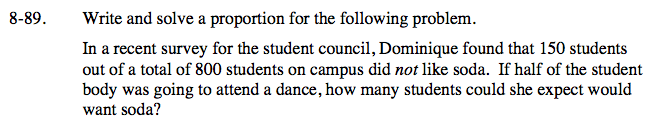### Home > CC2 > Chapter 8 > Lesson 8.3.3 > Problem8-89

8-89.
1. Write and solve a proportion for the following problem.

2. In a recent survey for the student council, Dominique found that 150 students out of a total of 800 students on campus did not like soda. If half of the student body was going to attend a dance, how many students could she expect would want soda? Homework Help ✎If 150 students dislike soda, how many students like soda?

800 − 150 = 650
650 students out of 800 like soda. How many students are attending the school dance?

$\text{If half of the student body is attending the dance, then: }\frac{800}{2} = 400$

400 students are attending the school dance. How many of those 400 students are expected to like soda?

Since 650 students out of 800 that like soda, we can set up a proportion to find the expected number of students at the dance that will like soda.

$\frac{650}{800} = \frac{x}{400} \text{ Solve for x.}$

x = 325 students